Dispatches from Class: How Your Website Boils Down to 1s and 0s

In Part I I'll explain how computers read 1s and 0s. Part II explains how software emerges from these numbers.

Most people know that 1s and 0s are the foundation for everything that happens inside a computer. What often isn’t so clear is what these 1s and 0s are actually doing. How does the text on your screen materialize from 1s and 0s? How can such complex applications–which include graphics, user interaction, you name it–all boil down to a string of binary?

To answer this question, we need to first look at the numbering system we’re all familiar with: base 10. When you read a number like 4012, you interpret its value based on the position of its parts: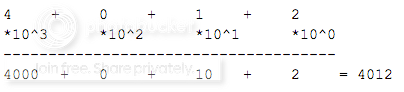It’s called base 10 because we’re multiplying each digit by a power of 10, then adding them up.

But what if we didn’t want to use 10? What would happen if we used, say, base 2?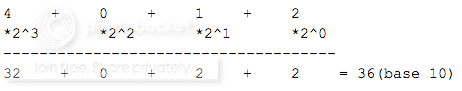To take the final step into binary we’ll restrict the digits we can use to only 1 and 0. This means instead of numbers like 15, 36, and 4, we’ll see numbers like 1111, 100100, and 100 respectively. Let’s look at an example up close: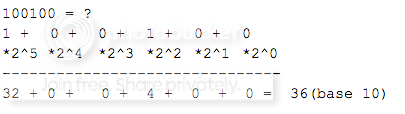So 100100 translates to 36. This is how computers store and read numbers internally. (This applies to positive integers. It gets a little more complicated with negatives and a lot more complicated with decimals. I’m happy to explain how those work if there’s interest.)

But of course you’re reading text, not a list of integers. Computers translate numbers to letters through a simple chart. The capital letter D, for example, maps to the number 68 in ASCII encoding. Here's a chart that shows the mapping for the full English alphabet. To spell my name in all capitals, we would say: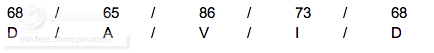Each letter corresponds to a unique number. Let’s convert those base 10 numbers to binary: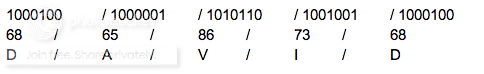So my name in binary (in ASCII encoding) is: 1000100 1000001 1010110 1001001 1000100.

When we save that value to a variable, we’re telling the computer to treat that sequence of numbers as a string of letters. We do this by declaring the variable as an array of characters (in statically typed languages), or by just putting quotations around the letters and letting the computer assume we’re saving letters (dynamically typed languages).

That exact same string of binary could mean something totally different depending on how we interpret it. Above we’ve assumed it maps to a string of letters, but we could also interpret it as a base 10 integer:

10001001000001101011010010011000100 = 18,391,344,324

The point is that the same string of binary can mean many different things, depending on how we choose to interpret the bits. This is how we can represent letters, words, integers, arrays, pretty much anything, using binary.

Now that we have a basic understanding of how computers store data, we’re set up to learn how different interpretations of these bits ends up as software. Check back for part II of this post, where we’ll build up arrangements of binary into a working program.

Update: Part II is live. See more blog posts from this series.